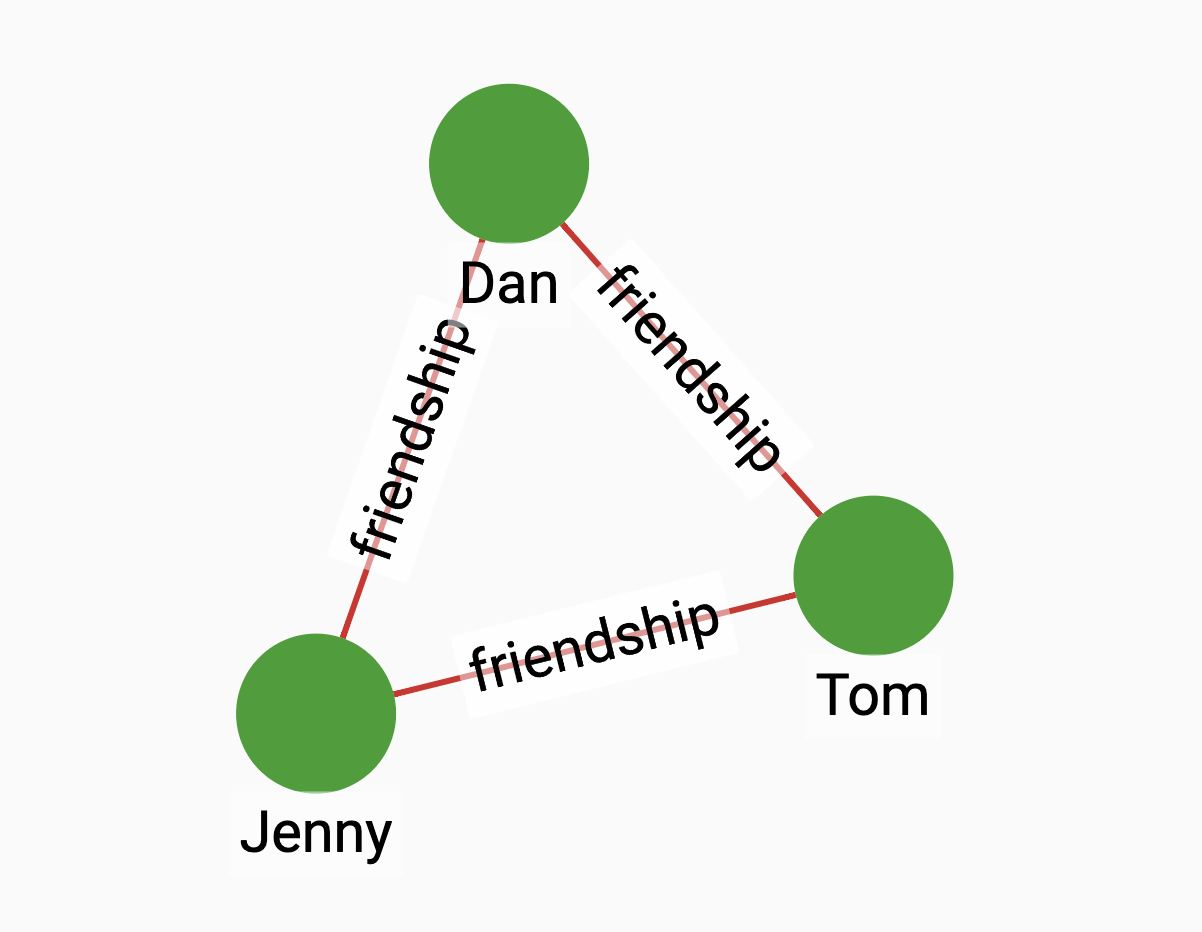The Adamic/Adar index is a measure introduced in 2003 by Lada Adamic and Eytan Adar to predict links in a social network, according to the number of shared links between two nodes. It is defined as the sum of the inverse logarithmic degree centrality of the neighbors shared by the two nodes.

${A(x,y)=\sum _{u\in N(x)\cap N(y)}{\frac {1}{\log {|N(u)|}}}}$

Where ${N(u)}$ is the set of nodes adjacent to u.

## Specification

``CREATE QUERY tg_adamic_adair(VERTEX a, VERTEX b, SET<STRING> e_type)``

### Time complexity

The algorithm has a time complexity of $O(D1 + D2)$, where D1 and D2 are the degrees of the two vertices.

## Parameters

Name Description Data type

`a`

A vertex.

`VERTEX`

`b`

A vertex.

`VERTEX`

`e_type`

Edge types to traverse.

`SET<STRING>`

## Return value

The Adamic Adar index between two vertices. If the two vertices do not have common neighbors, the algorithm will return a division by 0 error.

## Example

Suppose we have the graph below:Running the algorithm between Jenny and Dan will give us a result of $1/\log(2) = 3.32193$.

• Query

• Result

``RUN QUERY adamic_adar (("Jenny", "person"), ("Dan", "person"), ["friendship"])``
``````{
"error": false,
"message": "",
"version": {
"schema": 1,
"edition": "enterprise",
"api": "v2"
},
"results": [{"@@closeness": 3.32193}]
}``````